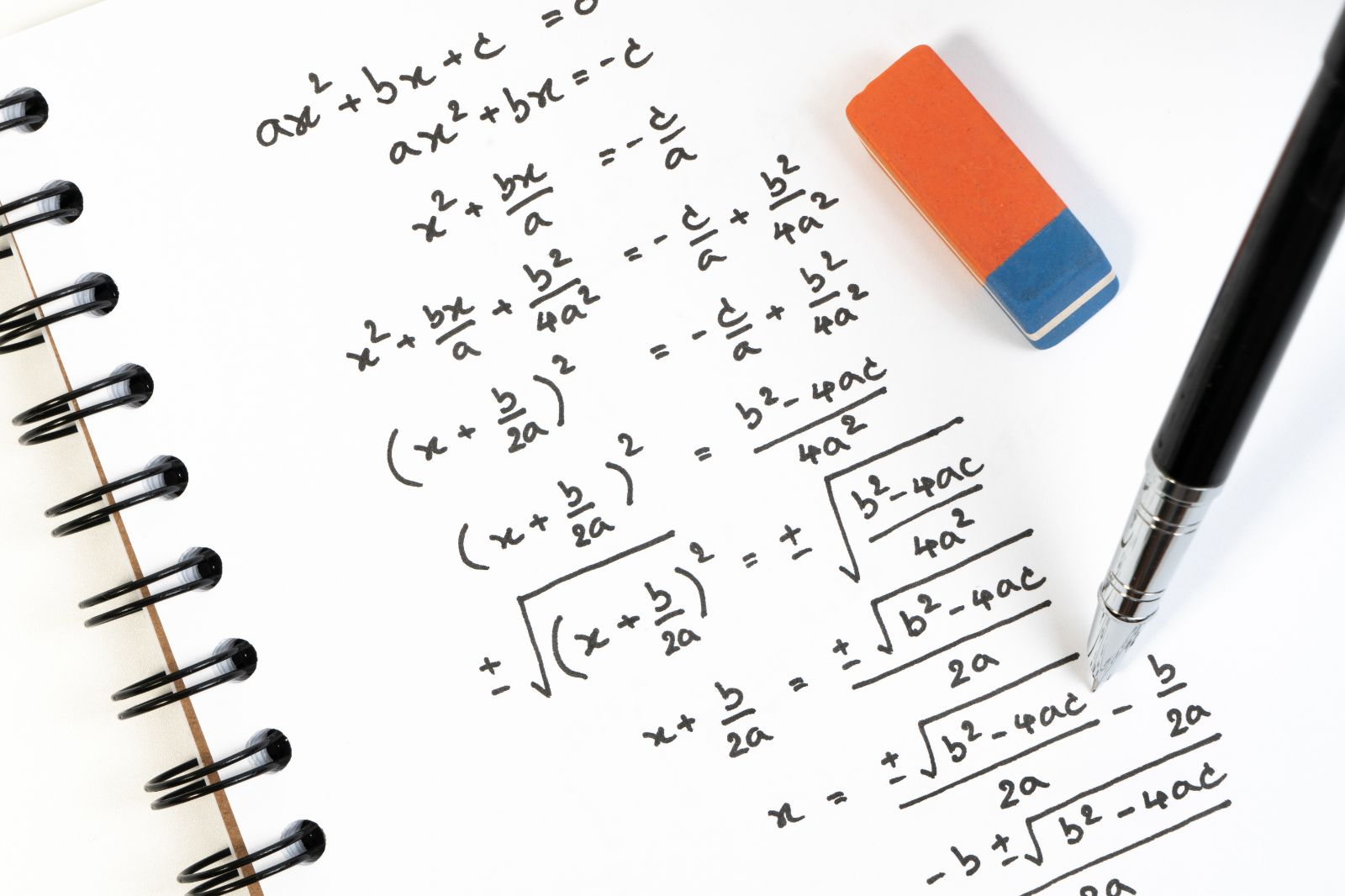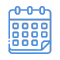# IGCSE Mathematics Exam Prep

A course that aids students in grasping complex Mathematics concepts for IGCSE success.From \$115

A course that aids students in grasping complex Mathematics concepts for IGCSE success.Level:Duration:
5 WeeksTest your knowledge on past papers and get detailed feedback from teachers.Access From Any Computer, Tablet or Mobile

This International GCSE Mathematics exam preparation course focuses on improving a student's understanding of mathematical theories as well as their confidence in performing mathematical calculations.

Access to an entire year’s Maths recorded live lessons allows the student to spend as much time on a concept as they need, and will provide them with the opportunity to truly understand the concepts with which they are struggling.

Each weekly assignment contains questions similar to those found in past papers so that students may become accustomed to the type of questions they can expect in their International GCSE examination.

Each assignment is marked by a teacher and individual feedback is given to each student. This ensures that each student is able to clearly identify where more attention is required.

After the assignments have been marked, students will be able to attend a Q&A session, where a teacher demonstrates how to solve each question. This will give the student a better understanding of how to best approach certain equations and questions.

## IGCSE Mathematics exam prep course topics

• Straight Lines Graphs: learn how to accurately draw straight-line graphs, solve equations graphically, work with points and lines as well as parallel lines, and more.
• Trigonometry: understand the Pythagoras Theorem, trigonometric ratios, calculating angles, and how to use sine, cosine, and tangent.
• Angle Properties: delve into angle properties, including angles of elevation and depression, as well as the sine and cosine of obtuse angles, and more.
• Number Sequences: understand how to find the nth term of a sequence, approach quadratic patterns, and more.
• Algebraic Manipulation: grasp the concept of algebraic manipulation through an understanding of expanding brackets, algebraic fractions, binomial and trinomial factorisation, and quadratic factorisation.

At the end of the mathematics exam preparation course, students will gain greater confidence in their mathematical ability.

## How the course works

Throughout the course, students will be provided with past exam papers designed to practice and reinforce the concepts covered in the Cambridge IGCSE Mathematics exams. Students will have the opportunity to ask questions and receive individualised feedback from the teacher during the online lessons.

Students will also receive a recording of each live lesson, to revisit should they wish to review a specific portion of the lesson.

The first 5 weeks of the course will focus on establishing which problem areas students are facing when completing past papers. After each assessment is completed, the teacher will work through problem areas that arose and provide detailed explanations to help students solve the problems.

The course concludes with a final mock exam, written in test conditions in an online lesson. In the final week, students will receive feedback from the teacher on their final exam and advice on how to prepare for the Cambridge IGCSE Mathematics exam.

## Why CambriLearn?

CambriLearn students have been achieving phenomenal results on their Cambridge exams for a decade. This is thanks to the individualised attention students receive from our experienced teachers, who provide feedback in a small online classroom environment.Duration:
5 WeeksCambridge aligned assignments to test your knowledge.Get detailed feedback on your work.Replay past lessons to strengthen your understandingAccess content from any device.

### Course scheduleStart Date:
28 August### Course Fees

\$115
For CambriLearn students
\$195
New students